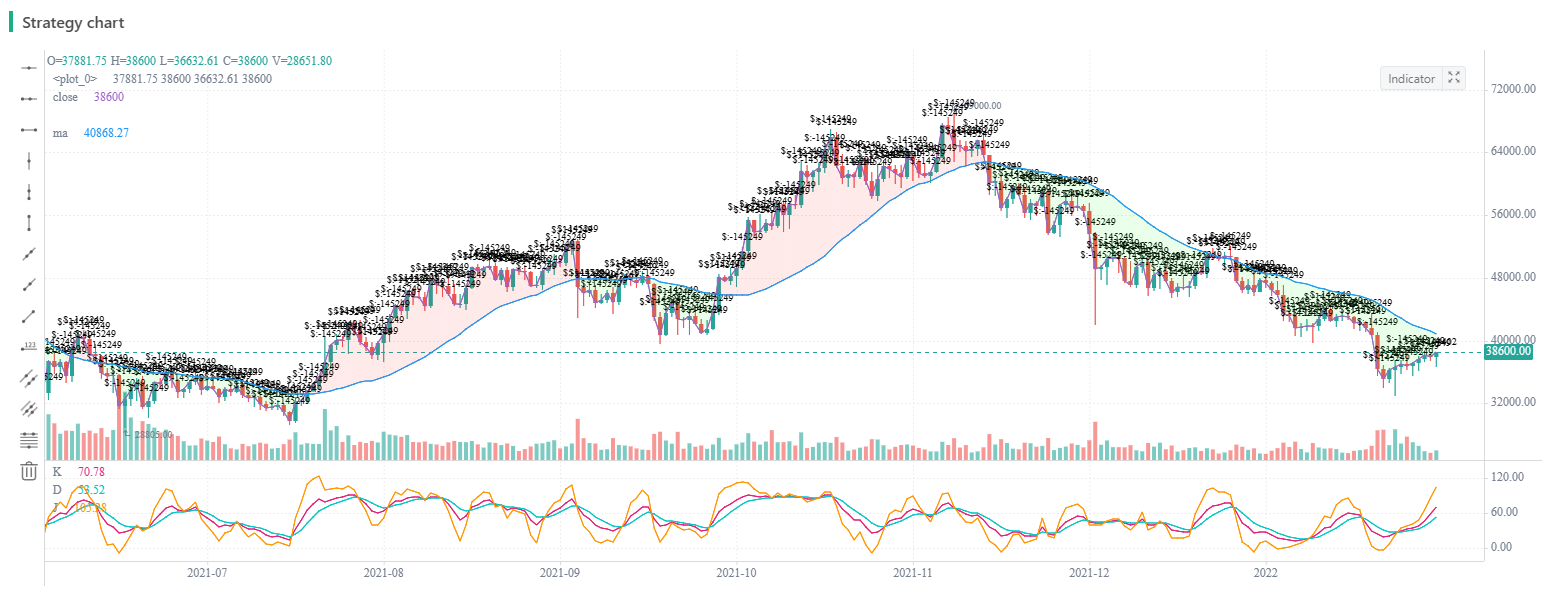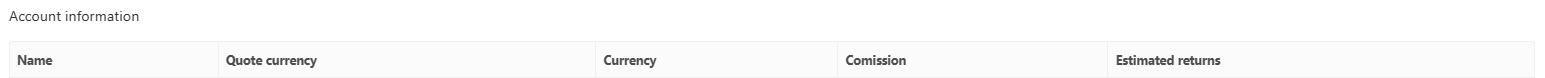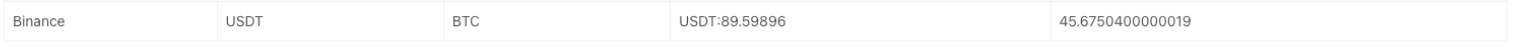Author: Lydia, Created: 2023-11-06 13:33:17, Updated:

Looking at a not-so-reliable trading idea – the K-line area trading strategy, in this article, we will explore the concept and try to implement the script.

### Main Idea of the K-Line Area Strategy

The K-line area strategy is a trading strategy based on the area relationship between price K-lines and moving averages. Its main idea is to predict possible trends in stock prices by analyzing the magnitude and changes of price trends, as well as shifts in buying and selling sentiment, thereby determining when to open positions and exit. This strategy relies on the area between the K-line and moving averages, as well as values from the KDJ indicator, to generate long and short trading signals.

### The Principle of K-Line Area Strategy

The area of the K-line refers to the spatial area between the price K-line and the moving average, calculated by subtracting the moving average value from each bar’s closing price and then summing it up. When there is a large increase in price over a long period of time, the K-line area will become larger, while during volatile markets or after volatility reversals, the K-line area is smaller. According to the principle of “what goes up must come down”, as an upward trend becomes larger and lasts longer, its corresponding K-line area also increases; thus increasing its probability for reversal - much like a spring that rebounds with greater force when stretched further. Therefore, setting a threshold for this K-line area can indicate when prices may have reached their peak and are likely to reverse.

To further confirm an impending trend reversal, we introduce the use of KDJ indicators which help determine shifts in buying or selling sentiment. The thresholds for the strategy and values for these indicators can be adjusted according to specific circumstances and needs in order to enhance accuracy.

### The Advantages of K-Line Area Strategy

The advantage of the K-line area strategy lies in its combination of the magnitude and changes of price trends, as well as the shift in buying and selling sentiment, providing a relatively complete quantitative trading strategy. Its advantages include:

• It provides a simple and intuitive method to identify the possibility of trend reversal, helping traders better grasp market trends.
• By combining the K-line area and KDJ indicator, it increases the reliability and accuracy of the strategy.
• High flexibility allows for parameter adjustments according to market conditions to meet different trading needs.

### Risk of K-line Area Strategy

Although the K-line area strategy has certain advantages, it also carries some risks, including:

• The setting of thresholds may require some experience and adjustment. If it set improperly, it could lead to misjudgment of market trends.
• The accuracy of the KDJ indicator is affected by market fluctuations and noise, which may result in false signals.
• The performance of the strategy may vary under different market conditions and needs constant optimization and adjustment.

### Optimization Direction of K-line Area Strategy

To optimize the K-line area strategy, consider the following directions:

• Parameter optimization: Continuously adjust and optimize threshold values and KDJ indicator parameters to adapt to different market conditions and trading needs.
• Risk management: Implement effective risk management strategies, including stop-loss and take-profit rules, to reduce loss risks.
• Multi-strategy combination: Combine the K-line area strategy with other strategies to improve the performance of comprehensive trading strategies.
• Real-time monitoring and adjustment: Regularly monitor the performance of strategies, adjusting and improving based on actual situations.

### Implement the Strategy Using JavaScript

• Calculate K-line Area

• Long position opening signal:

(1) The “K-line area” of the downward trend reaches the threshold, it can be established beforehand.

(2) KDJ indicator value is greater than 80.

• Short position opening signal:

(1) The “K-line area” of the upward trend reaches the threshold, it can be established beforehand.

(2) KDJ indicator value is less than 20.

• Exit for Long/Short positions: ATR trailing stop loss and take profit.

Code implementation

``````// Parameter
var maPeriod = 30
var threshold = 50000
var amount = 0.1

// Global variable
let c = KLineChart({})
let openPrice = 0

function calculateKLineArea(r, ma) {
var lastCrossUpIndex = null
var lastCrossDownIndex = null
for (var i = r.length - 1 ; i >= 0 ; i--) {
if (ma[i] !== null && r[i].Open < ma[i] && r[i].Close > ma[i]) {
lastCrossUpIndex = i
break
} else if (ma[i] !== null && r[i].Open > ma[i] && r[i].Close < ma[i]) {
lastCrossDownIndex = i
break
}

if (i >= 1 && ma[i] !== null && ma[i - 1] !== null && r[i - 1].Close < ma[i - 1] && r[i].Close > ma[i]) {
lastCrossUpIndex = i
break
} else if (i >= 1 && ma[i] !== null && ma[i - 1] !== null && r[i - 1].Close > ma[i - 1] && r[i].Close < ma[i]) {
lastCrossDownIndex = i
break
}
}

var area = 0
if (lastCrossDownIndex !== null) {
for (var i = r.length - 1 ; i >= lastCrossDownIndex ; i--) {
area -= Math.abs(r[i].Close - ma[i])
}
} else if (lastCrossUpIndex !== null) {
for (var i = r.length - 1 ; i >= lastCrossUpIndex ; i--) {
area += Math.abs(r[i].Close - ma[i])
}
}

return [area, lastCrossUpIndex, lastCrossDownIndex]
}

function onTick() {
var r = _C(exchange.GetRecords)
if (r.length < maPeriod) {
LogStatus(_D(), "Insufficient number of K-line")
return
}
var ma = TA.MA(r, maPeriod)
var atr = TA.ATR(r)
var kdj = TA.KDJ(r)
var lineK = kdj
var lineD = kdj
var lineJ = kdj
var areaInfo = calculateKLineArea(r, ma)
var area = _N(areaInfo, 0)
var lastCrossUpIndex = areaInfo
var lastCrossDownIndex = areaInfo

r.forEach(function(bar, index) {
c.begin(bar)
c.plotcandle(bar.Open, bar.High, bar.Low, bar.Close, {overlay: true})
let maLine = c.plot(ma[index], "ma", {overlay: true})
let close = c.plot(bar.Close, 'close', {overlay: true})
c.fill(maLine, close, {color: bar.Close > ma[index] ? 'rgba(255, 0, 0, 0.1)' : 'rgba(0, 255, 0, 0.1)'})
if (lastCrossUpIndex !== null) {
c.plotchar(bar.Time, {char: '\$:' + area, overlay: true})
} else if (lastCrossDownIndex !== null) {
c.plotchar(bar.Time, {char: '\$:' + area, overlay: true})
}
c.plot(lineK[index], "K")
c.plot(lineD[index], "D")
c.plot(lineJ[index], "J")

c.close()
})

if (tradeState == "NULL" && area < -threshold && lineK[lineK.length - 1] > 70) {
// long
}
} else if (tradeState == "NULL" && area > threshold && lineK[lineK.length - 1] < 30) {
// short
}
}

let stopBase = tradeState == "BUY" ? Math.max(openPrice, r[r.length - 2].Close) : Math.min(openPrice, r[r.length - 2].Close)
if (tradeState == "BUY" && r[r.length - 1].Close < stopBase - atr[atr.length - 2]) {
// cover long
openPrice = 0
}
} else if (tradeState == "SELL" && r[r.length - 1].Close > stopBase + atr[atr.length - 2]) {
// cover short
openPrice = 0
}
}

LogStatus(_D(), "area:", area, ", lineK[lineK.length - 2]:", lineK[lineK.length - 2])
}

function main() {
if (exchange.GetName().includes("_Futures")) {
throw "not support Futures"
}
while (true) {
onTick()
Sleep(1000)
}
}
``````

The strategy logic is very simple:

1. First, some global variables and parameters are defined, including:

Strategy parameters

• maPeriod: The period of moving average.
• threshold: A threshold used to determine the timing of buying or selling.
• amount: The quantity for each transaction.

Global variables

• c: A K-line chart object, used for drawing charts.
• openPrice: Records the opening price.

Calculate function

• calculateKLineArea function: It is used to calculate the area between the price and moving average line on a K-line chart over a certain period of time, and returns the area value, the index of the last upward crossing K-line, and the index of the last downward crossing K-line. These values are used in subsequent decisions to determine when to buy and sell.

Main loop function

• onTick function: It is the main strategy execution function, and here are the operations within the function:

a. Obtain the latest K-line data and ensure that the number of K-lines is not less than maPeriod, otherwise record status and return.

b. Calculate moving average line ma and ATR indicator atr, as well as KDJ indicator.

c. Get area information from areaInfo, last cross-over K-line index, and last cross-under K-line index.

d. Use K-line chart object c to draw K-lines and indicator lines while filling in different colors based on price’s relationship with moving average line.

e. Determine buying or selling timing according to conditions:

If tradeState is “NULL”, and the area is less than -threshold, and the K value of KDJ is greater than 70, execute a buy operation. If tradeState is “NULL”, and the area is greater than threshold, and the K value of KDJ is less than 30, execute a sell operation. f. Set stop loss and take profit conditions. If these conditions are met, close positions:

If it’s in buying state, when the price falls below the closing price of last trading day minus previous day’s ATR (Average True Range), close position. If it’s in selling state, when the price rises above last trading day’s closing price plus previous day’s ATR (Average True Range), close position.

main function: This serves as main execution entry point. It checks if exchange name contains “_Futures”. If so, an exception will be thrown; otherwise it enters into an infinite loop where onTick function gets executed every second.

In a word, this strategy mainly relies on K-line charts and technical indicators for making buying or selling decisions while also employing stop-loss & take-profit strategies to manage risk. Please note that this just serves as an example strategy which needs to be adjusted & optimized according to market situations & specific requirements during actual use.

On FMZ.COM, using JavaScript language didn’t require many lines of code, instead, it implemented this model easily. And with help from KLineChart function graphical representation of K-line chart area was easily achieved, too. The strategy design caters towards cryptocurrency spot markets by utilizing ‘Digital Currency Spot Trading Library’ template for placing orders through encapsulated functions within template, which makes it very simple & easy to understand and use.

### Strategy BacktestingI selected a backtesting period randomly. Although I didn’t lose money, I didn’t accumulate profits continuously, either, and the drawdown issue is quite significant. There should be other directions and room for optimization for the strategy. Those who are interested can try to upgrade the strategy.Through the strategy, we not only learned a rather unconventional trading idea, but also learned how to plot diagrams; representing the area enclosed by K-line and moving average line; ploting KDJ indicators etc.

### Summary

The K-line area strategy is a trading strategy based on price trend magnitude and the KDJ indicator. It helps traders predict market trends by analyzing the area between the K-line and moving averages, as well as shifts in buying and selling sentiment. Despite certain risks, this strategy can provide powerful trading tools through continuous optimization and adjustment, helping traders better cope with market fluctuations. Moreover, traders should adjust the parameters and rules of the strategy flexibly according to specific situations and market conditions to achieve better trading performance.

More